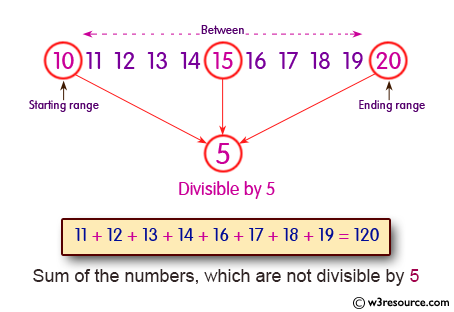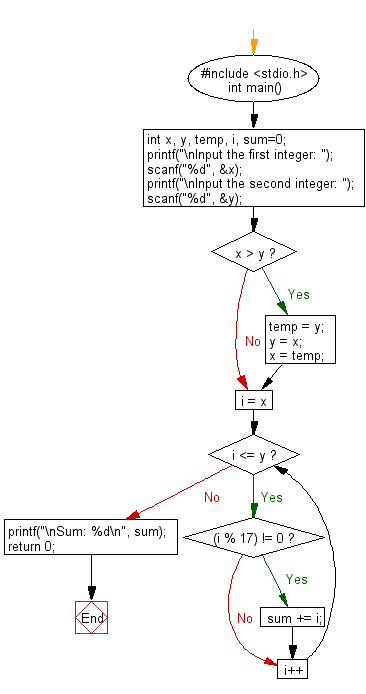﻿ C : Sum all numbers not divisible by 17 between two integers

# C Exercises: Calculate the sum of all number not divisible by 17 between two given integer numbers

## C Basic Declarations and Expressions: Exercise-39 with Solution

Write a C program to calculate the sum of all numbers not divisible by 17 between two given integer numbers.Sample Solution:

C Code:

``````#include <stdio.h>
int main() {
int x, y, temp, i, sum=0;

// Prompt for user input
printf("\nInput the first integer: ");
scanf("%d", &x);
printf("\nInput the second integer: ");
scanf("%d", &y);

// Swap values if x is greater than y
if(x > y) {
temp = y;
y = x;
x = temp;
}

// Calculate the sum of numbers not divisible by 17
for(i = x; i <= y; i++) {
if((i % 17) != 0) {
sum += i;
}
}

// Display the sum
printf("\nSum: %d\n", sum);

return 0;
}
``````

Sample Output:

```Input the first integer: 50

Input the second integer: 99

Sum: 3521
```

Flowchart:C Programming Code Editor:

What is the difficulty level of this exercise?

Test your Programming skills with w3resource's quiz.

﻿